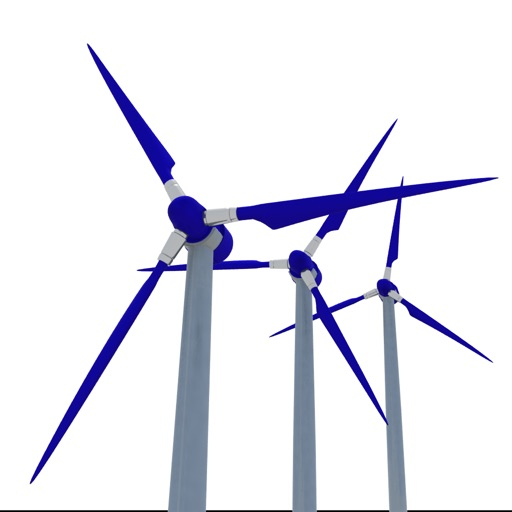## Fluid Mechanics Basics contains a large selection of Fluid Mechanics laws, equations, tables and reference material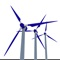# Fluid Mechanics Basics

by FPC Ltd.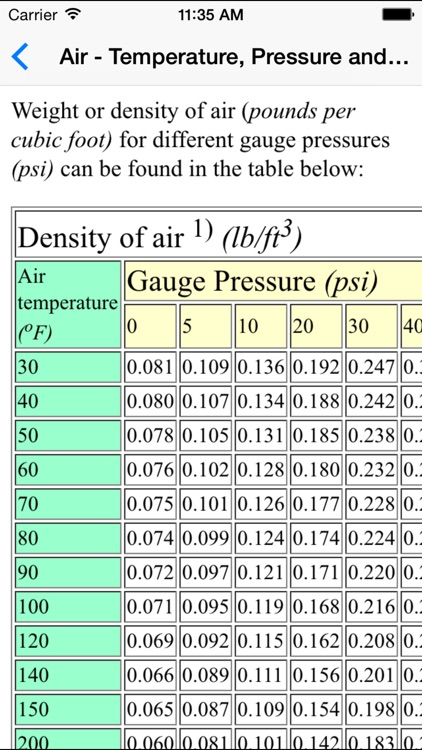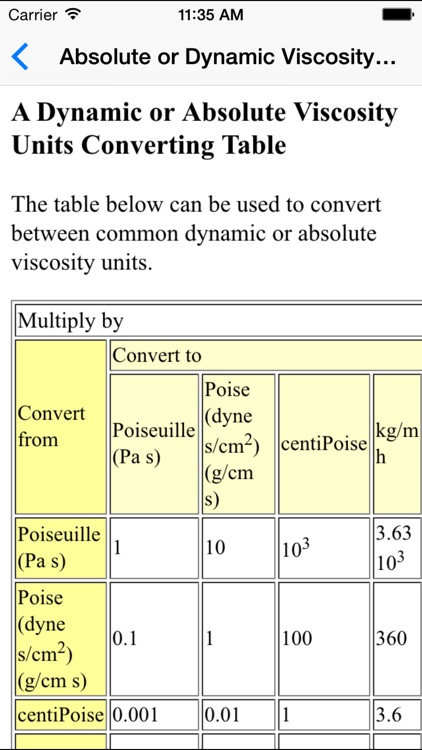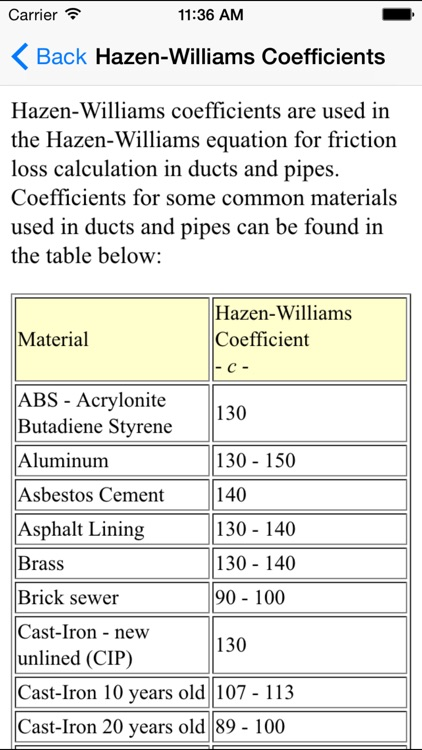Fluid Mechanics Basics contains a large selection of Fluid Mechanics laws, equations, tables and reference material.### App Details

Version
3.8
Rating
NA
Size
19Mb
Genre
Reference Education
Last updated
April 17, 2020
Release date
June 17, 2010

### App Screenshots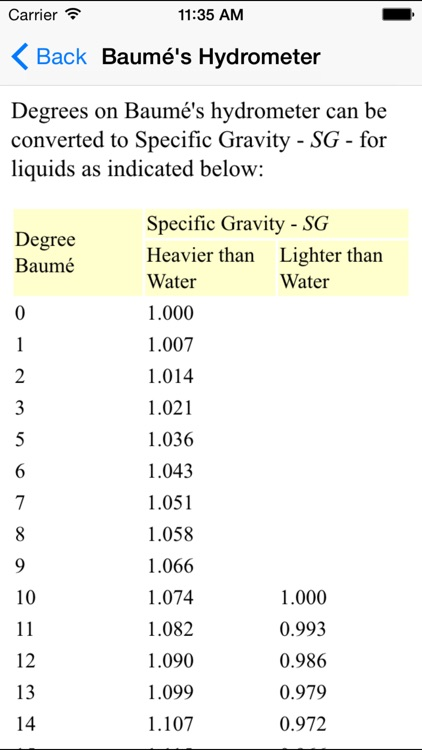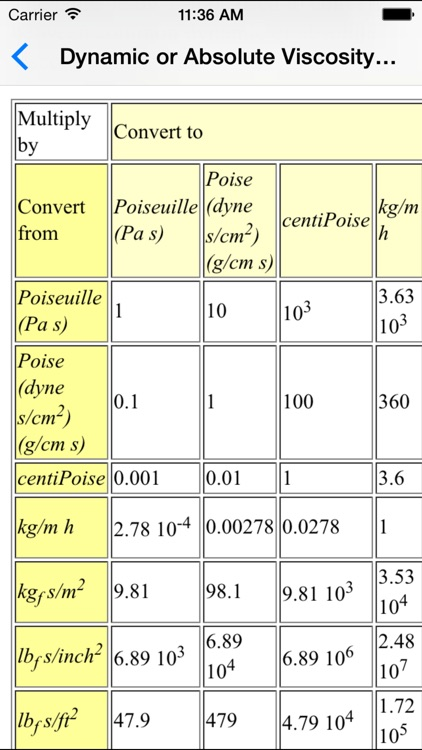### App Store Description

Fluid Mechanics Basics contains a large selection of Fluid Mechanics laws, equations, tables and reference material.

- Absolute or Dynamic Viscosity Converter table
- Air - Altitude, Density and Specific Volume
- Air - Temperature, Pressure and Density
- Baume's Hydrometer
- Bernoulli Equation
- Bulk Modulus and Fluid Elasticity
- Buoyancy
- Capillarity
- Cauchy Number
- Cavitation
- Centres of Gravity and Buoyancy
- Chezy Formula
- Colebrook Equation
- Compressible Gas Flow
- Conservation of Mass
- D'Arcy-Weisbach Equation
- Density, Specific Weight and Specific Gravity
- Drag Coefficient
- Dynamic Pressure
- Dynamic Viscosity
- Dynamic, Absolute and Kinematic Viscosity
- Energy and Hydraulic Grade Line
- Entrance Length and Developed Flow
- Equation of Continuity
- Equation of Mechanical Energy
- Equivalent Diameter
- Euler Number
- Flow Coefficient Cv and Flow Factor Kv
- Flow Section Channels
- Fluids (Kinematic Viscosities)
- Friction Coefficient at Laminar Flow
- Froude Number
- Gas Mixture Properties
- Gasses (Ratios of Specific Heat)
- Hazen-Williams Coefficients
- Hazen-Williams Equation
- Hydraulic Diameter
- Ideal Gas Law
- Imperial and SI Fluid Mechanics Dimensions and Units
- Isentropic Flow
- Kinematic Viscosity Converter Table
- Laminar, Transitional or Turbulent Flow
- Mach Number
- Major Loss in Ducts, Tube and Pipes
- Manning's Formula for Gravity Flow
- Manning's Roughness Coefficient
- Mass and Weight
- Minor Loss Coefficients for Duct Components
- Minor Loss Coefficients for Pipe and Tube Components
- Mixtures, Solutions and Suspensions
- Nozzles
- Oil Viscosity Conversion Values
- Orifice, Nozzle and Venturi Flow Rate Meters
- Pascal's Laws
- Pipe Velocities
- Poiseulle's Formula
- Prandtl Number
- Pressure
- Pressure Coefficient
- Pressure Units Converter Tables
- Pump Affinity Laws
- Reynolds Number
- Solubility
- Specific Work of Turbo Machines
- Speed of Sound Formulas
- Static Pressure and Pressure Head in Fluids
- Stoke's Formula
- Strouhal Number
- Total Pressure or Head Loss in Piple or Duct Systems
- U-Tube Manometer